﻿ An Algorithm for Automated Identification of Gust Fronts from Doppler Radar Data
 J. Meteor. Res.2018, Vol. 32Issue (3): 444-455PDF
http://dx.doi.org/10.1007/s13351-018-7089-7
The Chinese Meteorological Society
0

#### Article Information

YUAN, Yue, Ping WANG, Di WANG, et al., 2018.
An Algorithm for Automated Identification of Gust Fronts from Doppler Radar Data. 2018.
J. Meteor. Res., 32(3): 444-455
http://dx.doi.org/10.1007/s13351-018-7089-7

### Article History

Received June 14, 2017
in final form January 7, 2018
An Algorithm for Automated Identification of Gust Fronts from Doppler Radar Data
Yue YUAN1, Ping WANG1, Di WANG1, Huizhen JIA2
1. School of Electrical and Information Engineering, Tianjin University, Tianjin 300072;
2. Tianjin Bureau of Meteorology, Tianjin 300074
ABSTRACT: Gust fronts are weak narrow-band echoes of increased reflectivity at the background levels in the low-elevation fields of Doppler radar. An automated approach to gust front detection that relies on the image features of radar observations is presented in this paper. The algorithm is not sensitive to the variations in reflectivity values and gust front widths. The approach includes the following steps. First, a novel local binary with dual-template (LBDT) algorithm is designed as the fundamental algorithm to identify the potential areas of narrow-band echoes. Second, based on the disadvantages of the LBDT algorithm, several modifications are made, including splitting the intersecting lines, connecting the fragments, and filtering the edges and radial interference noise. Third, an optical flow method is used to determine whether a weak narrow-band echo is a gust front according to the prior knowledge that a gust front usually propagates in front of the associated generating storm. The results of experiments show that the proposed method can automatically identify gust fronts with a high probability of detection and a low false alarm rate. The automatic identification of gust fronts is potentially useful for accurate short-term weather forecasting, particularly in the forecasting of storm winds.
Key words: gust fronts     weak narrow-band echo     automated identification     local binary algorithm     intersection     optical flow method
1 Introduction

Gust fronts are generated by thunderstorms or squall line systems (Charba, 1974; Wakimoto, 1982). These gust fronts are followed by a surge of gusty winds on or near the ground and are often associated with a pressure jump, wind shift, temperature drop, and sometimes heavy precipitation (Bedard et al., 1977; Wilson and Schreiber, 1986; Quan et al., 2014). These fronts are also important for the initiation, growth, and decay of the thunderstorms (Wilson and Mueller, 1993; Wilson et al., 2004; Yu et al., 2012; Tao et al., 2014). Therefore, gust fronts are of interest in short-range forecasting and nowcasting. Because gust fronts have obvious correlations with thunderstorms and strong winds (Li et al., 2006; Qi et al., 2006) and have some indication to the development of thunderstorms, it is particularly necessary to develop high-quality methods for automatically identifying gust fronts.

However, the features of the gust fronts observed by Doppler radar are influenced by geographical factors, weather radar models, detection performance, scanning methods, and so on (Xu et al., 2016). For example, Troxel and Delanoy (1994) indicated that typical reflec-tivity values of gust fronts ranged from 5 to 20 dBZ, and the width seldom exceeded 3 km. Zheng et al. (2014) found that the range of intensity values was mainly between 5 and 30 dBZ, and the width was mainly from 2 to 10 km. Xu et al. (2016) demonstrated that the echo intensity of the gust fronts located near Nanjing city was generally between 0 and 21 dBZ, and the width was within 5–13 km. The various intensity values and widths have certain impacts on the previous algorithms.

Gust fronts and other convergence lines appear in a radar image as thin lines (Wilson and Schreiber, 1986; Smalley et al., 2005). In addition to the common general characteristics of convergence lines, a gust front usually propagates in front of the generating storm. This feature can be used to distinguish other convergence lines from a genuine gust front and reduce the false alarms. The reflectivity values of a gust front are generally larger than the values on both sides of the front. However, a gust front is sometimes obscured by other areas of clouds and precipitation, which results in disappearance of the difference between the gust front and its background. Therefore, an image segmentation method based on an edge and a region is not often useful in detecting gust fronts. Moreover, some interference echoes, such as radial interference noise, also appear as narrow bands on a radar map. Another phenomenon associated with automated gust front identification is that a gust front may be intersected by another weak narrow-band echo, and in such cases, the interferences may be ignored. In addition, a gust front presents a wind shear or a convergence line in the velocity field, but the recognition of the wind shear or the convergence line often failed due to the low quality Doppler radial velocity field from CINRAD (Zheng et al., 2014; Xu et al., 2016); so the radial velocity data are not considered to identify gust fronts in this paper. To sum up, the contradiction between the probability of detection (POD) and false alarm rate (FAR) can be a serious issue.

The purpose of this study is to automatically identify gust fronts in the reflectivity field of Doppler radar. This paper focuses on a variety of radar signatures of the above mentioned gust fronts. Thus, feature statistics and image processing techniques are applied in this work, and these methods are discussed in the following sections.

Section 2 contains a detailed description of the automatic identification algorithm of gust fronts. Section 2.1 describes the radar data and data pre-processing, followed by the gust front features in Section 2.2. Then, the proposed method, deemed the algorithm based on the local binary with dual-template (LBDT) method (LBDT-based for short), is constructed in Sections 2.3–2.6. Section 2.3 introduces the LBDT algorithm that is used to extract the potential areas of gust fronts. Section 2.4 concentrates on splitting the cases that have collided with other week narrow-band echoes and connecting fragments. Some interferences (i.e., the edges and radial interference noise) are filtered out in Section 2.5. Then, tracking is performed by using an optical flow method to determine whether the thin lines should be recognized as a gust front in Section 2.6. Section 3 addresses the gust front detection test and presents the results. The experiments in this section involve two sets of base data and an analysis of the failure cases. Finally, a summary of the results and the directions for future work are presented in Section 4.

2 Materials and method 2.1 Data and pre-processing

The radar base data from CINRAD are used in this paper. Two groups of radar data were selected to evaluate the performance of the algorithm. The first group contained 249 samples of gust fronts in 12 cases. Some were collected in Tianjin and others were provided by the Meteorological Observation Center of the China Meteorological Administration. The second group included 1680 images of radar data from 7 days with severe weather in June 2012 in Tianjin, China. The data contained 5 different types of gust fronts in 94 images.

Radar images are created by transforming radar base data from polar coordinates to Cartesian coordinates with nearest neighbor interpolation, and the resolution of an image is 1 km × 1 km in this study. In addition, the echo intensity value is divided into different intensity levels by reducing the intensity space, which is similar to the method proposed by Zheng et al. (2013). The method of reduction is given by Eq. (1):

 $Z' = \left\lfloor {Z/5} \right\rfloor \times 5,$ (1)

where Z ${\rm {and}} \,\, Z'$ are the original value and revised value of radar reflectivity, respectively, and $\left\lfloor x \right\rfloor$ represents the largest integer that is not greater than x. For example, the reflectivity value of 24 dBZ is modified to 20 dBZ according to Eq. (1).

2.2 The image features of gust fronts

Some examples of gust fronts are shown in Fig. 1, and the image features of the gust fronts (Delanoy and Troxel, 1993; Smalley et al., 2005; Zheng et al., 2014; Xu et al., 2016) can be summarized as follows:

1) Feature 1: A gust front generally appears in the front (including the right front and left front) moving side of a generating storm. In addition, the gust front is detached or partly separated from the generating storm (see Figs. 1a, b).

2) Feature 2 (width feature): The width of the range is mainly in the interval of 1 to 13 km.

3) Feature 3 (length feature): The length of the field is usually in the range of 40 to 300 km.

4) Feature 4 (variable reflectivity): At the same moment in time, the image values of a gust front can sometimes differ (Fig. 1d). In addition, the image values of a gust front usually change at various times (Figs. 1c, d); the times of Figs. 1c, d are 0931 and 0949 UTC, respec-tively, on 14 June 2006. However, the reflectivity values mainly range from 5 to 30 dBZ.

5) Feature 5 (ridge feature): The image values of a gust front are generally larger than the values on both sides of the front, forming a ridge feature. In some areas, the difference is small or non-existent.

6) Feature 6 (discontinuity): If the gust front is mixed with other echoes, it may be “polluted” and broken by areas of clouds and precipitation, as shown in Fig. 1e.

7) Feature 7 (intersection): A gust front may be intersected by another weak narrow-band echo (Fig. 1f).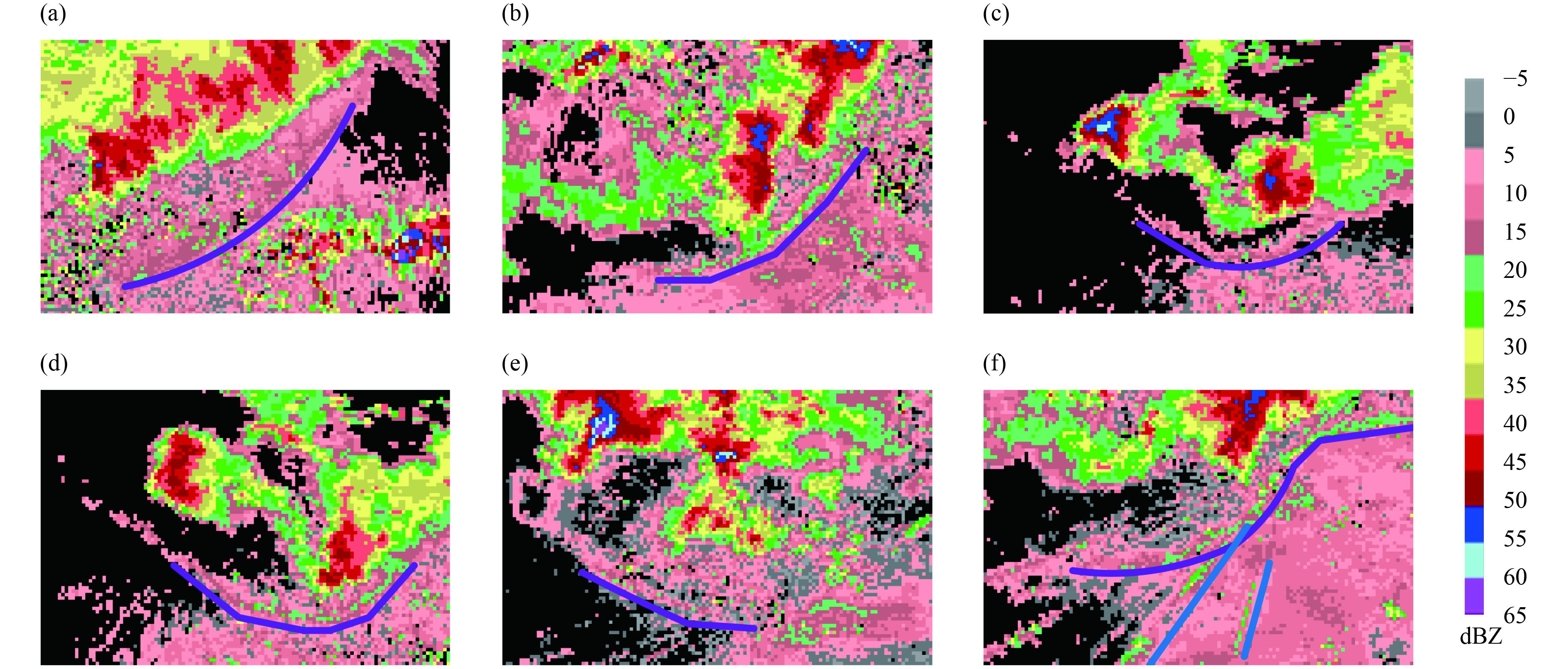Figure 1 Six examples of gust fronts in low-elevation reflectivity images. The gust fronts are marked with blueviolet lines, and other types of weak narrow-band echoes are denoted with blue lines (e.g., Fig. 1f).
2.3 The local binary with dual-template (LBDT) algorithm

First, according to Feature 4 of a gust front, the reflectivity values in the ridge area are generally in the interval from 0 to 35 dBZ; and gust fronts appear as weak ridge regions in reflectivity images (Feature 5). The gust front is set as the foreground, and the area on its two sides is set as the background. Therefore, the values of the points in the foreground are equal or close to those around them and larger than those in the background. Hence, the value of a point in the gust front should be greater than the mean of the surrounding points. Second, the width of a gust front is mainly within the range of 1–13 km. Due to the difference in width of gust fronts, two templates of different sizes should be considered. In this paper, the LBDT method is proposed to identify the potential areas of gust fronts that are not sensitive to varying width and reflectivity values of gust fronts. Additionally, the target gust front should have a sufficiently large length.

The LBDT method is based on the convolution calculation given in Eq. (2):

 $\left\{\!\! {\begin{array}{*{20}{c}} {\begin{array}{*{20}{c}} {{g_1}(i,j) = \displaystyle\sum\limits_{s = - n}^{s = n} {\sum\limits_{t = - n}^{t = n} {{w_1}(s,t)f(i + s,j + t),\quad \quad \quad } } }& {{w_1}(s,t) =\displaystyle \frac{1}{{{{(2n + 1)}^2}}}} \end{array}} \\[14pt] {\begin{array}{*{20}{c}} {{g_2}(i,j) =\displaystyle \sum\limits_{s = - (2n + 1)}^{s = 2n + 1} {\sum\limits_{t = - (2n + 1)}^{t = 2n + 1} {{w_2}(s,t)f(i + s,j + t),} } }& {{w_2}(s,t) =\displaystyle \frac{1}{{{{(4n + 3)}^2}}}} \end{array}} \end{array}} \right.,$ (2)

where f(i, j) denotes the reflectivity value at point pij, gk(i, j) (k = 1, 2) represents the result of a convolution operation to determine the average value of the region, wk(s, t) (k = 1, 2) is the convolution kernel, and n decides the sizes of the two templates. According to the width of the gust front, the parameter n is selected as 3. Two different convolution templates are shown in Fig. 2. If the reflectivity value of the black grid (a point) is larger than the mean of the black and gray rectangles (corresponding to g1 and g2, respectively), the point can be considered a point in the gust front. If the width of the gust front is greater than the black template, it is suggested that the value of a point in the middle of the gust front is equal to the mean of the black rectangle (g1) and is larger than the mean of the gray rectangles (g2). Based on the analysis above, the LBDT algorithm for weak ridge region detection is designed as follows:

Step 1: For every point pij in a reflectivity image

Step 1.1: bool = 0;

Step 1.2: if f (i, j) does not belong to [0, 35], go to Step 1.4;

Step 1.3: calculate the convolution of point pij by Eq. (2); if f (i, j) ≥ g1(i, j) and f (i, j) > g2(i, j), bool = 1; else, go to Step 1.4;

Step 1.4: if bool = 1, the point is set as a foreground point; else, the point is set as a background point.

Step 2: Calculate the length of every foreground region (narrow band); if the length is less than the threshold L1 (e.g., 15 km), this region is considered a spot and is part of the background.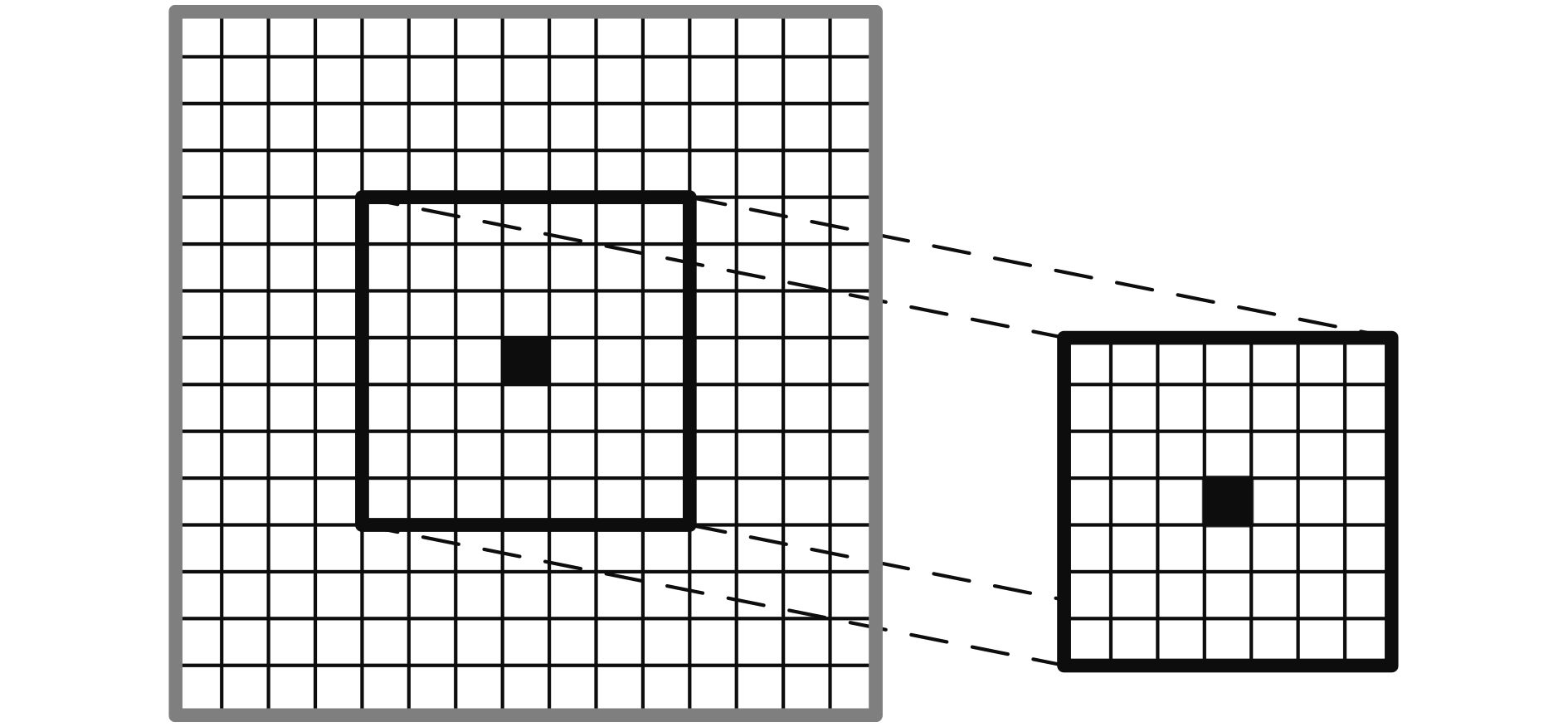Figure 2 Convolution templates. Each grid in the figure represents an 1 km × 1 km area. The black grid represents the center of the template. In addition, the black and gray rectangles represent two convolution templates with different sizes.

The LBDT algorithm can extract gust fronts. However, at the same time, the obtained foreground regions include non-target areas, such as edges, weak narrow-band echoes (except for gust fronts), and radial interference noise. As a result, some methods must be designed based on the other features of gust fronts (including Features 1, 6, and 7) to filter out the non-target areas.

With the white line representing the extraction area, Fig. 3 shows examples of the results of the LBDT operation. The figure shows not only the gust fronts (Figs. 3b, f) but also several types of simultaneous interference as follows:

(1) Edges: The white line in Fig. 3a is called the class of interference C1;

(2) The ridge region intersecting the gust front: The white branching line in the lower right portion of Fig. 3b is called the class of interference C2;

(3) Radial interference noise: The white line in Fig. 3c is referred to as the class of interference C3; and

(4) The weak narrow-band echo (except for gust fronts): The white lines in Figs. 3d, e are called the class of interference C4.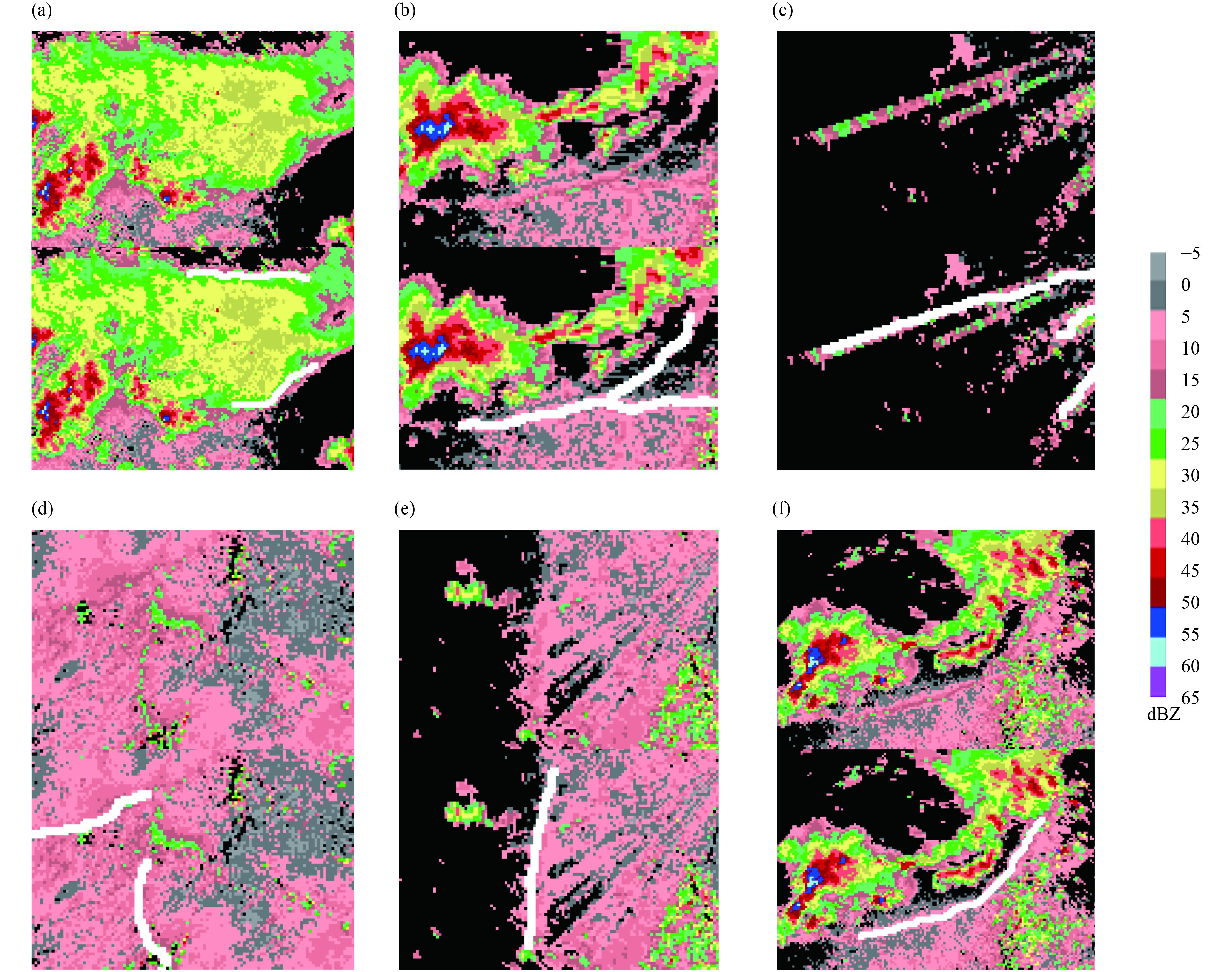Figure 3 Typical cases of the foreground regions extracted by the LBDT algorithm. In each group of images, the upper image shows the original image, and the white lines in the lower image represent the foreground regions extracted by the LBDT algorithm.
2.4 Splitting and connecting

A gust front is shown as a smooth narrow band without intersections or turns in a radar reflectivity image. However, the targets detected by the LBDT algorithm may be affected by the class of interference C2 of the gust fronts or broken areas. Because a gust front and other narrow-band echoes can intersect each other, the gust front must be split. In the case that a gust front is broken into two or more parts, it is necessary to connect them. In addition, the intersection points indicate that the gust fronts converge with other boundary layer convergence lines, where severe weather is likely to occur (Abulikemu et al., 2015, 2016; Xi et al., 2015).

To separate or connect the foreground regions obtained in Section 2.3, the regional structure must be described. A skeletonization algorithm is an effective method for representing the two-dimensional regional structure. To reduce the impact of small burrs on the contour of the region, a smooth deburring process of the regional border is needed. Then, the series of points can be obtained in the skeleton image (Lam et al., 1992; Spitzner and Gonzalez, 2015).

2.4.1 Splitting

To separate the class of interference C2 from genuine gust fronts, three types of points, endpoints, intersection points, and turning points, are identified in the skeleton image. The turning point is the point in the curve that is not derivable. In other words, the right derivative of the point and the left derivative of the point in the curve are not equal. The intersection point or turning point indicates that there may be a collision of two weak narrow-band echoes in that location. These points are the keys to skeleton separation.

(1) Turning point recognition: For a point pi on the curve, the angle between vector ${\overrightarrow {{{\text{p}}_i}{\text{p}}} _{i - \tau }}$ and vector ${\overrightarrow {{{\text{p}}_i}{\text{p}}} _{i + \tau }}$ is calculated, where points pi-τ and pi+τ on the curve are on different sides of point pi. If the angle is far from 180°, the point pi is considered as a turning point.

(2) Endpoint and intersection point recognition: The endpoints and intersection points can be detected by using the local binary pattern (LBP) operator (Ojala et al., 1996; Liu et al., 2014). In a binary image after skeletonization, a foreground point pi with a value equal to 1 can be classified as an endpoint, an intersection point, or neither, by determining the boundary value distribution of the 3 × 3 and 5 × 5 regions surrounding it. Specifically, if the boundary point value in the 5 × 5 region is 1, and not connected to the regional center within the range of the 5 × 5 region, such as the gray grid shown in Fig. 4c, the value must be set to 0. Then, the 8 and 16 binary chain codes are generated to describe the distribution of the value of the zone boundary that begins in the upper left corner of the two regions and continues along the regional border in the counterclockwise direction. The numbers of changes to 0 and 1 at point pi are recorded by n3|pi and n5|pi, respectively.

If n3|pi = 2, as shown in Fig. 4a, point pi is an endpoint; If n3|pi ≥ 6 or n5|pi ≥ 6, as shown in Fig. 4b, point pi is an intersection point.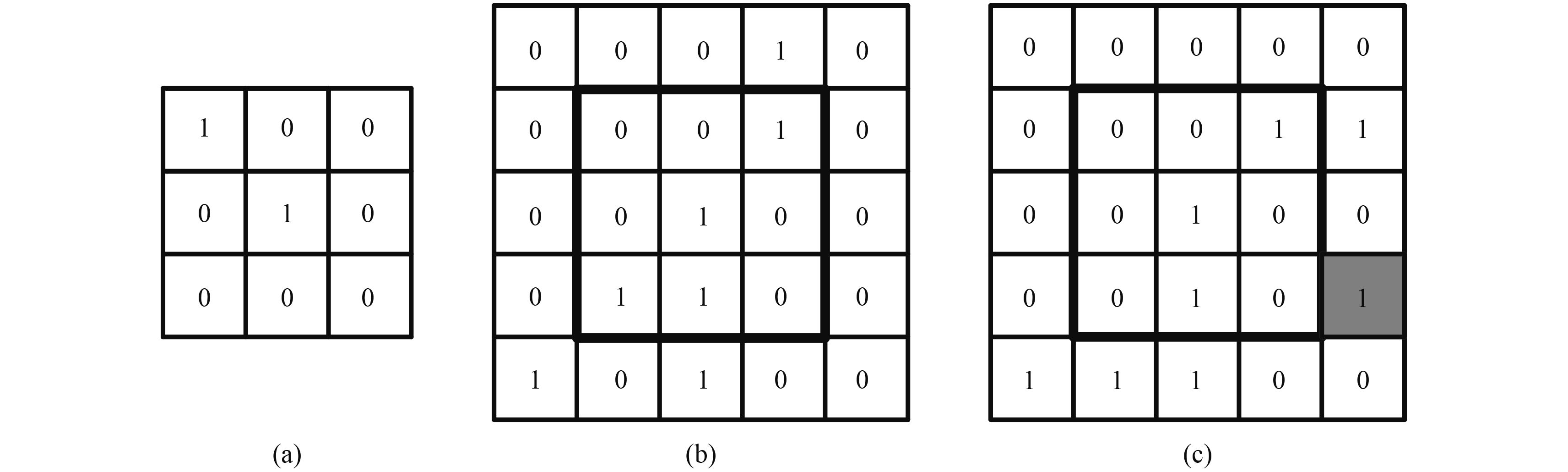Figure 4 The local skeleton regions. Each grid in the figure represents an 1 km × 1 km area. The value 1 indicates a foreground point on the thin line extracted by the LBDT algorithm, and 0 is a background point.

(3) The skeleton is separated at the intersection points and turning points. Then, these points become new endpoints.

2.4.2 Connecting

As discussed above, the lines broken in Section 2.4.1 and the fragments detected by the LBDT algorithm may need to be connected. If the directions of the two lines are similar and the gap between the two lines is not large, they are likely two parts of a line. Therefore, the connection conditions of endpoint A of curve li and endpoint B of curve lj (see Fig. 5) can be set as Eq. (3):

 $\left\{ {\begin{array}{*{20}{c}}{\cos ({\overrightarrow {{\rm{BA}}} },{{{\theta }}_{\rm{A}}}) < \cos ({\alpha }_1)}\\[8pt]{\cos ({\overrightarrow {{\rm{AB}}} },{{{\theta }}_{\rm{B}}}) < \cos ({\alpha }_2)}\\[8pt]\!\!{\cos ({{{\theta }}_{\rm{B}}},{{{\theta }}_{\rm{A}}}) < \cos ({\alpha _3})}\\[8pt]{| {\overrightarrow {{\rm{AB}}} } | < {d_1}}\end{array}} \right.,$ (3)

where θA and θB are vectors in the direction of line prolongation (see Fig. 5), αk (k = 1, 2, 3) is the angle threshold (e.g., 150°) and d1 is the distance threshold (e.g., 25 km).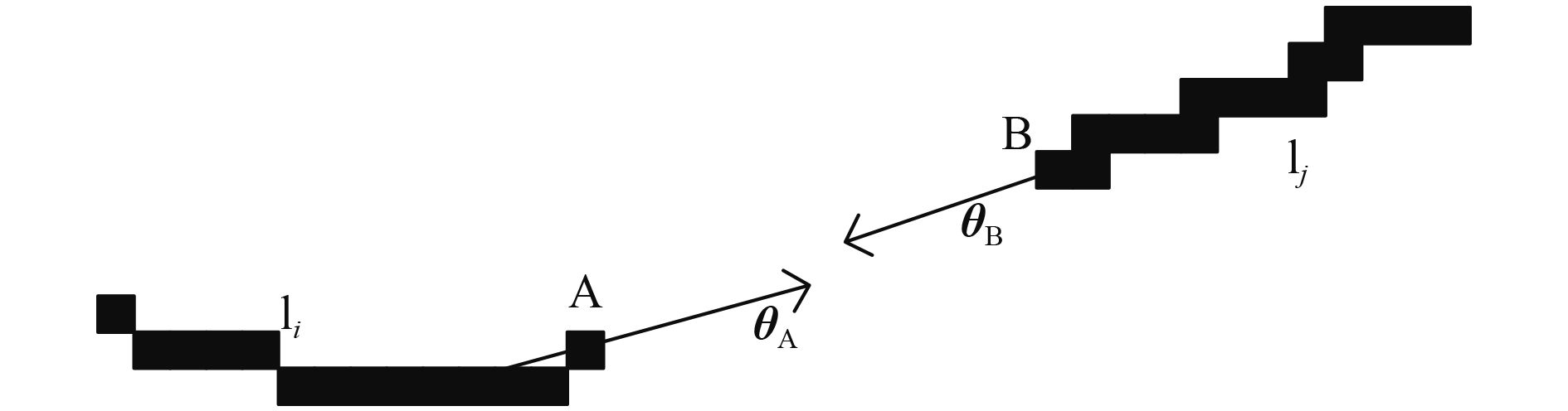Figure 5 Illustration of the endpoints and their directions of line prolongation.

If point A and a plurality of points are in line with the connection rules, the minimum value of cos(θB, θA) is selected as the successful match point B. Namely, points A and B are on the same curve. To ensure the reasonableness of the connection, this step should not be executed if the line crosses the high-reflectivity region (greater than or equal to 40 dBZ) or low-reflectivity region (less than –5 dBZ). In addition, by taking into account Feature 3 of a gust front, any thin line with a length of less than 20 km is also filtered out.

2.5 Filtering edges and radial interference noise

In this step, principal component analysis (PCA) (Wold et al., 1987) is performed to decompose these curves and filter the edges and radial interference noise. Conceptually, the PCA results for two-dimensional data provide two principal components. The vector direction of the largest variance is deemed the first principal component axis and defined as vector e1, which can be used to approximate the curve direction. The second principal component axis, defined as vector e2, is perpendicular to the first component and can be used to find points on both sides of the curve.

2.5.1 Filtering edges

The reflectivity values of the foreground region identified by the LBDT algorithm may not be larger than the values on both sides. These regions appear as the edges of areas of clouds and precipitation and are called the class of interference C1, as shown in Fig. 3a. Overall, the values of the weak narrow-band echo (including gust fronts) are larger than those on both sides, but not for every point on the border. The edge (the class of interference C1) values are only larger than those on one side. According to Feature 2 of a gust front, the distance from the background to the curve (the middle line of the weak narrow-band echo) is 2–6 km. The method of rejecting the class interference C1 of a gust front is shown as follows:

Step 1: Apply PCA to the curve to obtain the unit vector e2.

Step 2: The distance k varies from 2 to 6 km, and the variable αk is initialized to 0. For every point pi, where i = 1, 2, ..., m, on the curve, the corresponding coordinates of point qi on one side of the curve can be obtained by qi = pi + ke2, where pi and qi denote the coordinates of points pi and qi in Cartesian coordinates, respectively. Then, every αk is calculated by using Eq. (4):

 $\left\{ {\begin{array}{*{20}{c}}{\begin{array}{*{20}{c}}{{\alpha _{{k}}} = {\alpha _{{k}}}},\,\,\,\quad\quad & f|{{\text{p}}_i} > f|{{\text{q}}_i};\quad\,\end{array}}\\[8pt]{\begin{array}{*{20}{c}}\!\!\!\!\!\!\!\!{{\alpha _{{k}}} = {\alpha _{{k}}} + 1},&{ f|{{\text{p}}_i} = f|{{\text{q}}_i}};\end{array}}\\[8pt]{\begin{array}{*{20}{c}}\!\!\!\!\!\!\!{{\alpha _{{k}}} = {\alpha _{{k}}} + 2},\,&{ f|{{\text{p}}_i} < f|{{\text{q}}_i}},\qquad\end{array}}\end{array}} \right.i = 1, 2, ..., m,$ (4)

where ${f|{\text{p}}_i}\,\, {\rm{ and }}\,\, f|{{\text{q}}_i}$ represent the reflectivity values at point pi and point qi, respectively.

Step 3: The standardization α0 is obtained with Eq. (5):

 ${\alpha _0} = \frac{{\min ({\alpha _k})}}{m}.$ (5)

Step 4: Similarly, k is equal to –2, –3, –4, –5, and –6, and a weighting ratio β0 is obtained for the other side.

Step 5: If α0 < Th and β0 < Th (e.g., Th = 0.75), the curve is considered a weak narrow-band echo; if these conditions are not satisfied, the curve is considered the class interference C 1 of the gust front and should be filtered.

2.5.2 Filtering radial interference noise

After the operations above, the thin lines (weak narrow-band echoes) that include the gust fronts are extracted. The flowchart of thin line extraction (Sections 2.3–2.5) is shown in Fig. 6.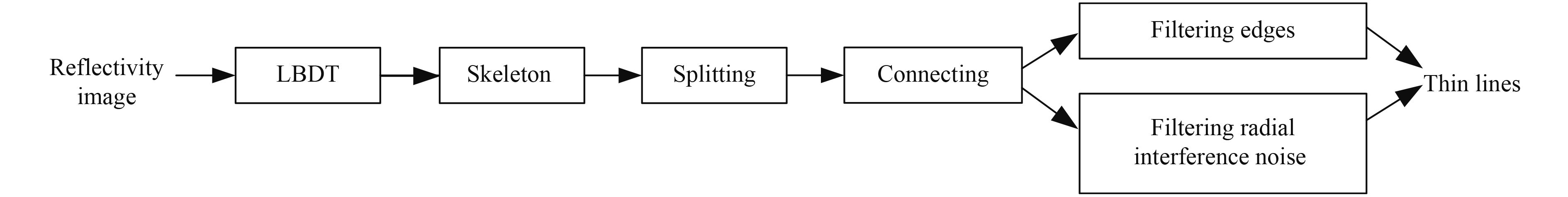Figure 6 The flowchart of the process presented in Sections 2.3–2.5
2.6 Tracking based on optical flow 2.6.1 Optical flow

Farnebäck (2003) proposed a method based on the polynomial expansion of optical flow for time-series grayscale images. Additionally, the principle for estimating the moving speed of the previous time point pi in the last image was described. In the last image, assuming the coordinate of point pi is x, the gray value function of the neighborhood O(x) of point pi can be approximately represented by a quadratic polynomial ${f_1}({x}) = {{x}^{\rm{T}}}{{A}_{1}}{x} +$ ${b}_1^{\rm{T}}{x} + {{{c}}_1}$ (with coordinates as the independent variables and the value of the coordinate as the dependent variable in the function). In this image, the value function of the region O(x) can be approximately represented by a quadratic polynomial ${f_2}\left( {x} \right) = {{x}^{\rm{T}}}{{A}_{{2}}}{x} + {b}_{2}^{\rm{T}}{x} + {{c}_{2}}$ . The approximation discussed above refers to substituting the coordinates and values of every point in region O(x) and fitting the corresponding coefficients using a weighted least squares method. Assuming that point pi moves from x to x + d, Eq. (6) can be constructed for region O(x),

 $\begin{split}{f_2}({x}) = {f_1}({x} - {d}) & = {({x} - {d})^{\rm{T}}}{{A}_1}({x} - {d}) + {b}_1^{\rm{T}}({x} - {d}) + {{c}_1} \\ &= {{x}^{\rm{T}}}{{A}_2}{x} + {b}_2^{\rm{T}}{x} + {{c}_2}.\end{split}$ (6)

Based on the corresponding coefficients, which are equal, the movement vector d of point pi between two time steps can be determined.

Through this method, the speed of every point in the radar image can be calculated from the images of the current and previous times. Only two types of points are considered in the radar image: points that consist of the weak narrow-band echo detected above and points that consist of the storm cell and are located in the high-reflectivity region (greater than or equal to 40 dBZ). Thus, for the small deformation of a storm at two adjacent times, the speed of the storm is approximated as the average speed of all points in the storm. Similarly, the speed of the gust front is approximated as the average speed of every point in the gust front.

2.6.2 Identifying gust fronts

In fact, a gust front appears stable in the low-elevation reflectivity image in its cycle. In addition, the front usually satisfies the following three propagation guidelines, which are useful for filtering out interference class C4. 1) The moving direction of the gust front and the orientation of the gust front are approximately perpendicular to each other; 2) The gust front is usually located at the moving front (including the right front and left front) side of the generating storm; and 3) The gust front either propagates at a speed similar to the generating storm or moves away from the generating storm. However, not all gust fronts comply with the three propagation guidelines. For example, in some rare cases, gust fronts come from the backside of storms. This algorithm is likely to fail in these cases.

The previous historical information is a more effective method for identifying gust fronts, compared with that using the three propagation guidelines. If a thin line in historic information corresponds to a gust front, it can be directly identified as the gust front without considering the three propagation guidelines. This relation requires that the new location of the gust front determined from the prior history of the optical flow field is coincident or similar to the position of the thin line in the current time step. In short, the process of gust front identification can be described as follows. If a prior history event for a thin line exists, the thin line can be declared a gust front. Alternatively, if the thin line follows the three propagation guidelines above, it can also be declared a gust front.

The prior historic information of gust fronts is also conducive to the optimization of the thin lines that represent the gust fronts. In addition, the results of the optical flow method can be used for prediction purposes (Cao et al., 2015; Chen et al., 2017). For example, Fig. 7 illustrates the tracking and prediction of a gust front in successive radar reflectivity images. As discussed above，the optical flow field can be calculated by two successive radar reflectivity images (Figs. 7a, b). Therefore, the tracking result can be obtained by calculating the overlap ratio of the minimum circumscribed rectangles of the blue and white lines in Fig. 7b. The prediction result can be obtained based on the estimated motion vectors shown as the blue line in Fig. 7c.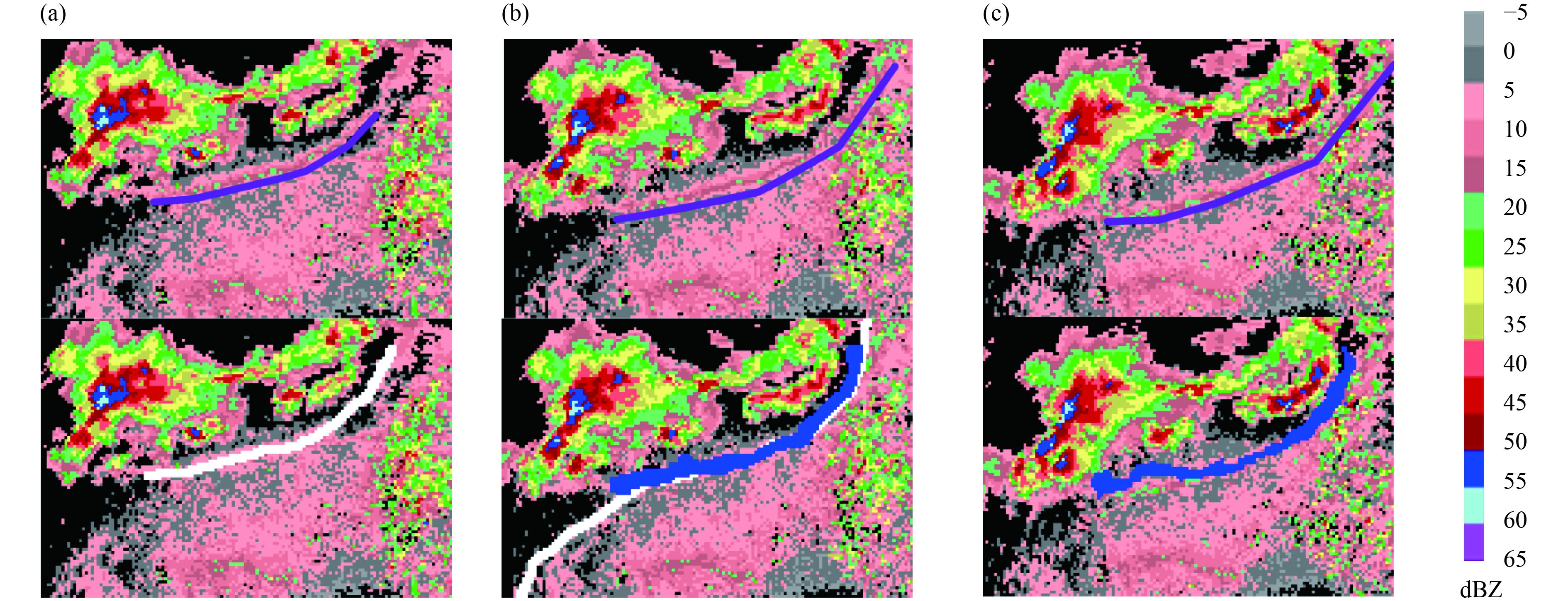Figure 7 Illustration for tracking and predicting a gust front in successive radar reflectivity images. The gust fronts are denoted by blueviolet lines in the upper images. The white lines in lower images of Figs. 7a, b are the detected thin lines. The blue line in Fig. 7b shows the new location of the detected line in Fig. 7a calculated based on the optical flow field. The blue line in Fig. 7c represents the predicted gust front.
3 Experiments 3.1 Evaluation metrics

The POD, FAR, and critical success index (CSI) are used to evaluate the automated detection algorithm. The formulas are as follows:

 ${\rm {POD}} = \frac{a}{{a +c}},\quad\,\,$ (7)
 ${\rm {FAR}} = \frac{b}{{a + b}},\quad\,\,$ (8)
 ${\rm {CSI}} = \frac{a}{{a + b + c}}.$ (9)

The three equations are calculated by using an ordinary 2 × 2 table (Table 1). In this paper, an “observed yes” indicates that there is a gust front in the radar data which is determined by the weather forecaster; an “observed no” indicates that there is no gust front; an “identified yes” indicates that the algorithm outputs a line (gust front); and an “identified no” indicates that the algorithm outputs no line. Therefore, “a” is the number of identified gust front samples obtained if the detected lines are fully or partially (more than 50%) in the gust front; “b” is the number of erroneously identified samples obtained if the detected lines are completely outside the gust front; and “c” is the number of unidentified gust front samples obtained if there are no detected lines in the gust front.

Table 1 A typical 2 × 2 contingency table
 Observed yes Observed no Identified yes a b Identified no c d

The POD indicates how many samples can be detected in the real gust fronts, and FAR indicates how many samples are incorrect in the identified gust fronts. The two metrics are complementary. In addition, the CSI takes both of these factors into account. Because the objective of this paper is to generate an algorithm that can predict average behaviors for the automated identification of gust fronts, the applied method should be resistant to outlier behavior. In the evaluation results, the POD should be as large as possible, and the FAR should be as small as possible. The three evaluation metrics range from 0 to 1. If the POD equals 1 and the FAR equals 0, the performance is perfect. Overall, if the CSI equals 1, the method exhibits optimal performance.

3.2 Results

Two groups of radar data were selected for analysis in this paper and the effect of the LBDT-based algorithm presented in Section 2 was assessed. In these two sets of tests, the total number of base data (TBD), the number of gust front samples (GFS), the number of identified gust front samples (IGFS), and the number of erroneously identified samples (EIS) were counted. The POD, FAR, and CSI were calculated to evaluate the performance of the proposed algorithm.

In the first test, the performance of the LBDT-based algorithm was compared with another automatic identification algorithm of gust fronts for CINRAD: the algorithm proposed by Zheng et al. (2014) (Zheng’s algorithm for short). The identification results of 12 cases of gust fronts are described in Table 2. According to Table 2, the CSI value for the LBDT-based algorithm is 0.848 as compared to 0.511 for Zheng’s algorithm. The LBDT-based algorithm has a relatively higher POD value of 0.875 than Zheng’s algorithm with POD value of 0.751. As discussed above, the FTC method of Zheng’s algorithm sometimes failed to identify the gust fronts. For example, Zheng’s algorithm missed detection some samples for the data of Shijiazhuang radar on 26 July 2011. In addition, it should be noted that the POD for Zheng’s algorithm in this paper is lower than the POD value of 0.879 in Zheng et al. (2014). This may be caused by different samples, poor velocity data quality, or different thresholds. The LBDT-based algorithm achieves a FAR of 0.035, which is much lower than 0.385 for Zheng’s algorithm, because there are many false alarms generated by radial interference noise (e.g., white lines shown in Fig. 8a) and other boundary layer convergence lines (e.g., white lines in Fig. 8b) in Zheng’s algorithm.

Table 2 The number of samples and sample identification for the first test
 Date Radar site GFS LBDT-based Zheng’s IGFS EIS IGFS EIS 20050613 Tianjin 10 9 0 10 6 20050614 Tianjin 26 23 1 19 13 20050709 Tianjin 20 17 1 13 8 20050711 Tianjin 29 23 0 21 13 20060614 Tianjin 17 15 0 13 6 20060624 Tianjin 17 16 2 14 12 20090603 Zhengzhou 22 20 2 18 17 20100706 Lianyungang 16 15 2 14 9 20110726 Shijiazhuang 18 17 0 10 8 20110729 Beijing 21 19 0 15 14 20110730 Changzhou 29 24 0 28 5 20120516 Yancheng 24 20 0 12 6 Total 249 218 8 185 117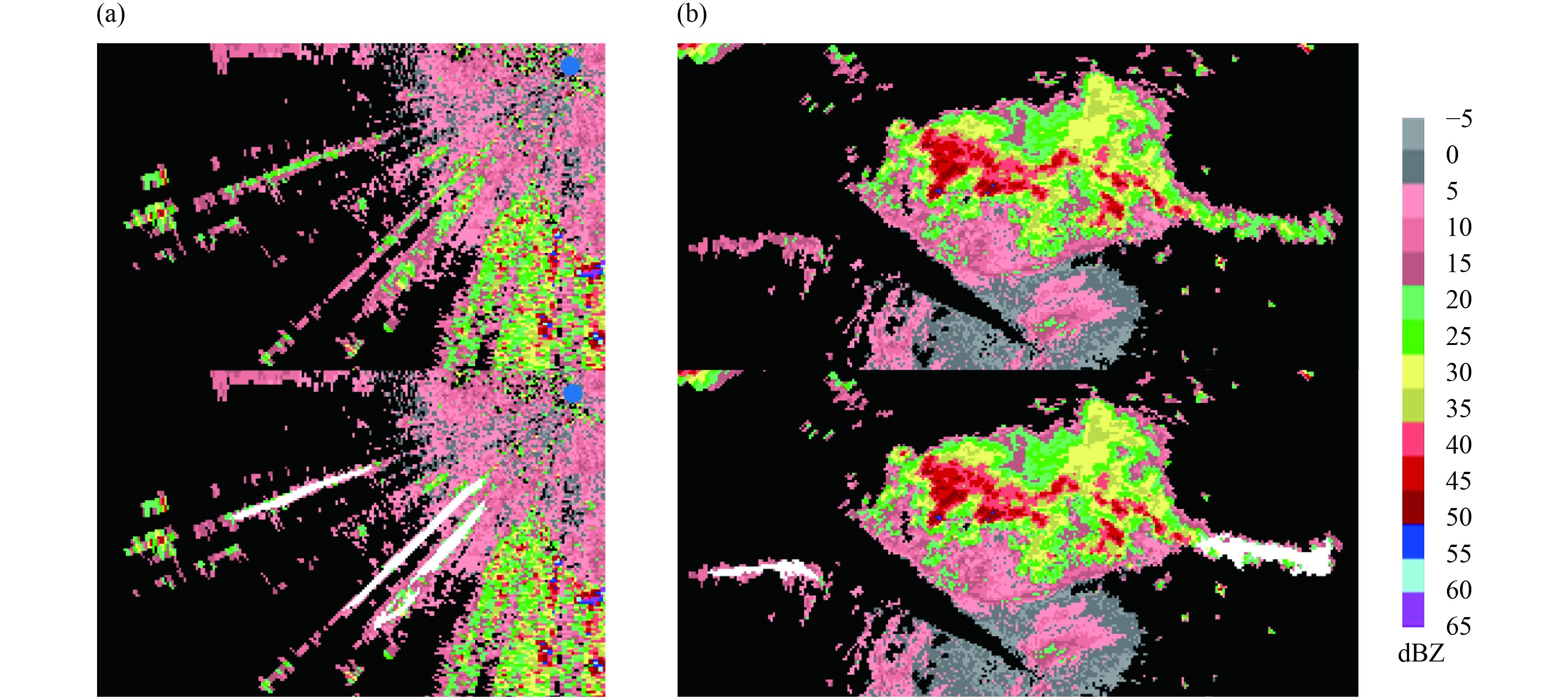Figure 8 Several typical false alarms by Zheng’s algorithm. The upper image shows the original image, and the white lines in the lower image represent the recognition results by Zheng’s algorithm. The blue dots in Fig. 8a show the location of the radar.

In addition, a large number of images without gust fronts were used in the second test set to investigate the FAR of the LBDT-based algorithm. There were 7 cases of severe weather in June 2012 in Tianjin, including 5 cases of gust fronts. During a period of 7 days (1680 radar data images), a gust front appeared in 94 images, and no gust front was observed in 1586 images. Notably, 74 of the 94 gust fronts were detected by the proposed algorithm. However, 22 false gust fronts were also detected. According to Table 3, based on the results of the second test, the POD is 0.787, FAR is 0.229, and CSI is 0.638. Gust fronts were detected in all five cases, but the test result of one case is worse than the results of the other four because the gust front was obscured by areas of clouds and precipitation. Compared with the first test, the POD and CSI are reduced, and the FAR increases. However, the statistical results of these samples are similar to the actual results detected by this algorithm during operation.

Table 3 The number of samples and sample identification for the second test
 Date TBD GFS IGFS EIS 20120603 240 12 3 3 20120606 240 12 8 3 20120607 240 0 0 2 20120609 240 31 28 5 20120610 240 0 0 0 20120621 240 14 13 4 20120624 240 25 22 5 Total 1680 94 74 22

Several detection results of gust front for the LBDT-based algorithm are exemplified in Fig. 9. Figure 9a shows a typical identified gust front. Figure 9b shows the final recognition result of Fig. 3b after removing the class of interference C2. Figures 9c and 9d show two false positives. Some echoes (e.g., white line in Fig. 9c) that meet the conditions of gust front features were mistakenly identified as gust fronts. The curves (e.g., white line in Fig. 9d) are connected by several laterally aligned spots that were improperly identified as weak narrow-band echoes. Figures 9e, 9f, and 9g show three types of unrecognized cases. The gust front is not obvious in Fig. 9e, and the gust front is mistaken for radial interference noise in Fig. 9f. There are several small spots in the image of the gust front in Fig. 9g, which is why the gust front could not be identified by the LBDT algorithm presented in Section 2.3.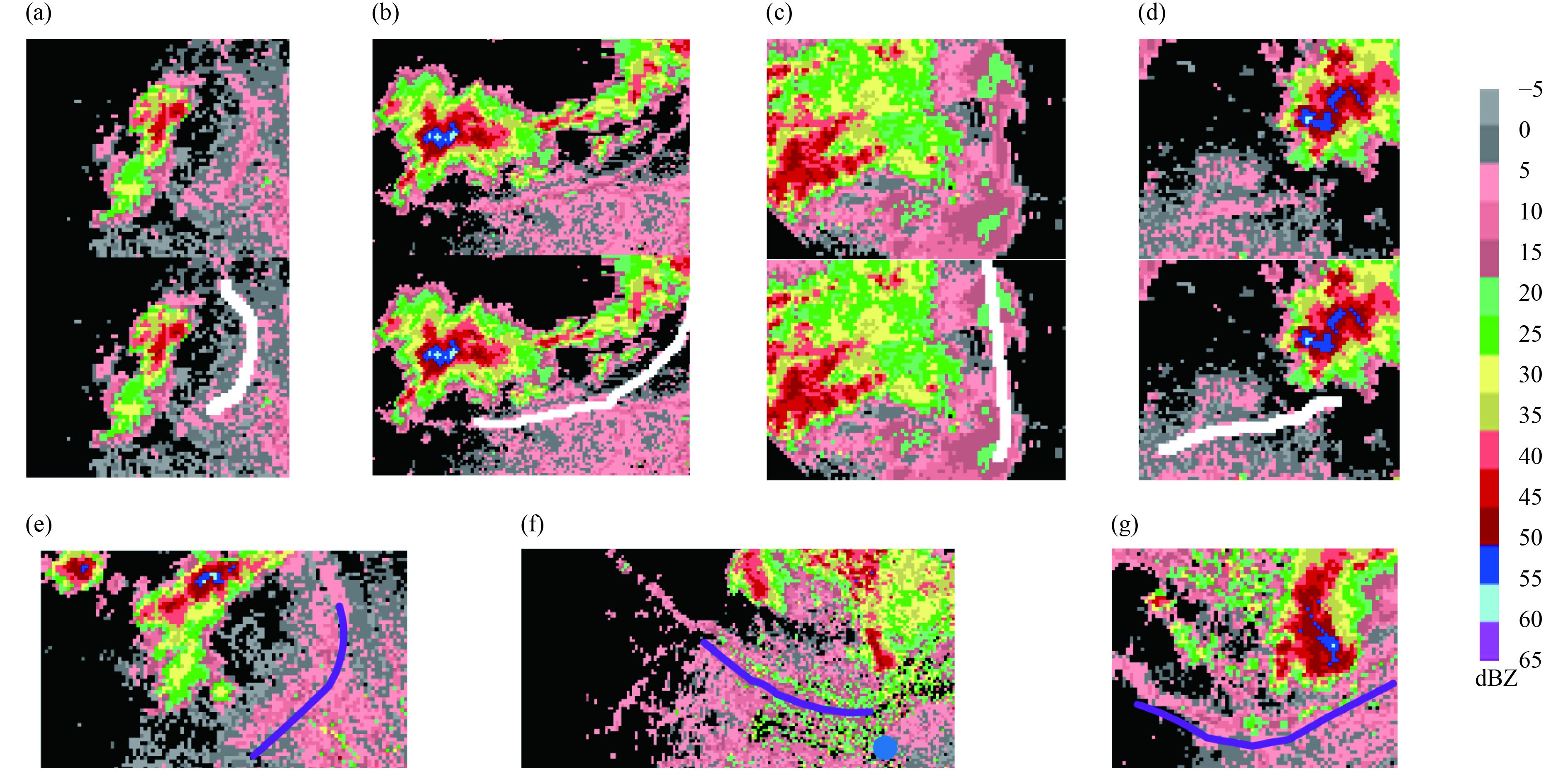Figure 9 Several typical cases of recognition results for the LBDT-based algorithm. The upper image shows the original image, and the white lines in the lower image represent the recognition results in Figs. 9a–d. The blueviolet lines denote the unrecognized gust fronts in Figs. 9e–g. The blue dot in Fig. 9f shows the location of the radar.
4 Conclusions

Based on the statistical feature analysis and image processing techniques, a method for automatically detecting gust fronts from radar data, deemed the LBDT-based algorithm, is proposed in this study. The main contributions of this study are as follows. First, the algorithm is not sensitive to the variations in reflectivity values or gust front widths in the extraction of potential gust front areas. Second, the skeleton splitting operation based on intersection points and turning points is very effective if several gust fronts and other narrow-band echoes collide; specifically, the intersection may supply the initiation point of a storm. Third, a gust front is usually located at the front moving side of a strong convective system, and this characteristic is used to separate other convergence lines from the genuine gust front and reduce false positives. Finally, a tracking algorithm for gust fronts is developed by using an optical flow method. However, the proposed method has some limitations, as discussed in Section 3.2, and these issues should be resolved in the future.

The LBDT-based algorithm is evaluated based on two groups of Doppler radar data, and the results indicate that this method can automatically identify gust fronts with a high POD and a low FAR. The LBDT-based algorithm can be modified easily for other radar types, such as rain radar, because this method only utilizes reflectivity imagery. In addition, this gust front recognition system could provide assistance for the analysis of storms and winds. This system provides a foundation for further research regarding the automatic identification of boundary layer convergence lines and the quantitative relations of storm winds and gust fronts.

Acknowledgments. We thank the Meteorological Observation Center of the China Meteorological Administration for providing the radar base data and Bai Li for providing assistance with this work.

References